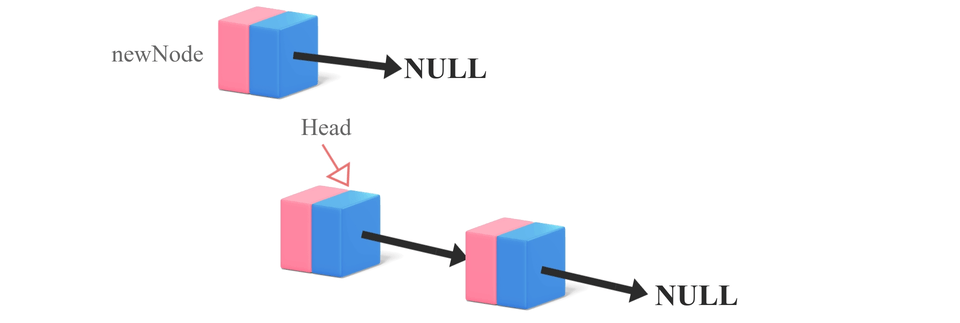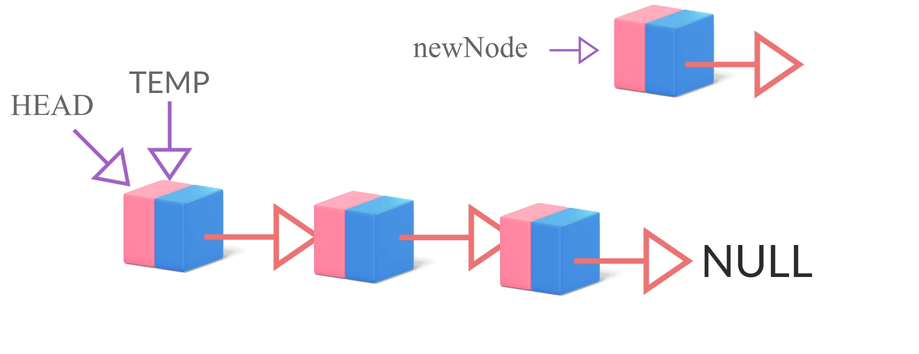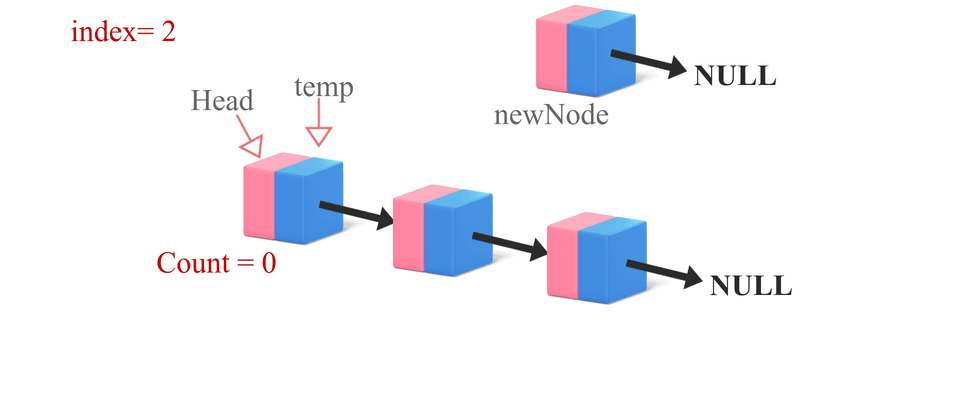# Insertion Operations in a Linked ListCreated June 15, 2021

Now that we've learned how the basic operations on a linked list are done let's look at the different types of insertion in a linked list. There are three ways of node insertion in a linked list.

## The Three Ways of Node Insertion

We can insert a node in the following ways:

### 1.Insertion from the front end

The front-most node of a linked list is called the head. Through this method, we add the elements such that they replace the head. This insertion function goes something like this -

-> Create a newnode.

-> Check if the list is empty or not. If so, make the head point at the newnode.

-> Otherwise, make newnode's next to point at the head. Then make the head point at newnode. Following is the C code for the same:

``````NODE *FrontInsertion(int data, NODE *head){
NODE *newnode = createNode(data);
}
else{
}
}
``````

The insertion operation looks something like this -### 2.Insertion from the back end

We've already discussed this type of insertion here. In this form of insertion, we simply traverse all the way to the end of the list and then append the newly created node. The steps for insertion are- -> Create a newnode.

-> Check if the list is empty or not. If so, then make the head point at the newnode.

-> Otherwise, using 'temp' variable traverse all the way to the end of the list.

-> Next of the last node is made to point at the newnode. The C code for the same is -

``````NODE *BackInsertion(int data, NODE *head){
NODE *newnode = createNode(data);
}
else{
while(temp->next != NULL){
temp = temp->next;
}//temp reaches end of the linked list;
temp->next = newnode;//the node that was previously pointing to NULL
// is now pointing at the newnode;
}
}
``````

The process looks something like this -### 2.Insertion at a specific Index Value

This method is a little bit trickier than the other two. We'll be using a counter variable to implement this form of insertion. Following are the steps- -> Create a newnode.

-> Set a counter variable value to 0.

-> A temp variable is set equal to the head of the list.

-> Traverse through the list using the temp variable, increment the counter by 1 each time.

-> When the counter is equal to 1 less than the target index value, set the next of the newnode to the next of the node temp is pointing at. -> Then set the next of temp to the newnode.

The C code for the same looks like this:

``````NODE *InsertatIndex(int data, NODE *head, int index){
NODE *newnode = createNode(data);
int count=0;
while(count != index-1){
temp = temp->next;
count++;
}
//temp reaches the index before the target index
newnode->next = temp->next;
temp->next = newnode;

Illustration of the whole process: